# Calculator multiplying a fraction by a whole number

The calculator performs basic and advanced operations with fractions, expressions with fractions combined with integers, decimals, and mixed numbers. It also shows detailed step-by-step information about the fraction calculation procedure. Solve problems with two, three, or more fractions and numbers in one expression.

### 6 * 3/4 = 9/2 = 4 1/2 = 4.5

Spelled result in words is nine halfs (or four and one half).

### How do you solve fractions step by step?

1. Multiple: 6 * 3/4 = 6 · 3/1 · 4 = 18/4 = 9 · 2/2 · 2 = 9/2
Multiply both numerators and denominators. Result fraction keep to lowest possible denominator GCD(18, 4) = 2. In the next intermediate step , cancel by a common factor of 2 gives 9/2.
In words - six multiplied by three quarters = nine halfs.

#### Rules for expressions with fractions:

Fractions - use the slash “/” between the numerator and denominator, i.e., for five-hundredths, enter 5/100. If you are using mixed numbers, be sure to leave a single space between the whole and fraction part.
The slash separates the numerator (number above a fraction line) and denominator (number below).

Mixed numerals (mixed fractions or mixed numbers) write as non-zero integer separated by one space and fraction i.e., 1 2/3 (having the same sign). An example of a negative mixed fraction: -5 1/2.
Because slash is both signs for fraction line and division, we recommended use colon (:) as the operator of division fractions i.e., 1/2 : 3.

Decimals (decimal numbers) enter with a decimal point . and they are automatically converted to fractions - i.e. 1.45.

The colon : and slash / is the symbol of division. Can be used to divide mixed numbers 1 2/3 : 4 3/8 or can be used for write complex fractions i.e. 1/2 : 1/3.
An asterisk * or × is the symbol for multiplication.
Plus + is addition, minus sign - is subtraction and ()[] is mathematical parentheses.
The exponentiation/power symbol is ^ - for example: (7/8-4/5)^2 = (7/8-4/5)2

The calculator follows well-known rules for order of operations. The most common mnemonics for remembering this order of operations are:
PEMDAS - Parentheses, Exponents, Multiplication, Division, Addition, Subtraction.
BEDMAS - Brackets, Exponents, Division, Multiplication, Addition, Subtraction
BODMAS - Brackets, Of or Order, Division, Multiplication, Addition, Subtraction.
GEMDAS - Grouping Symbols - brackets (){}, Exponents, Multiplication, Division, Addition, Subtraction.
Be careful, always do multiplication and division before addition and subtraction. Some operators (+ and -) and (* and /) has the same priority and then must evaluate from left to right.

## Fractions in word problems:

• Equivalent fractionsAre these two fractions equivalent -4/9 and 11/15?
• Fractions 3Calculate 1/9 of 27:
• CompareCompare fractions ?. Which fraction of the lower?
• Write 3Write a real world problem involving the multiplication of a fraction and a whole number with a product that is between 8 and 10 then solve the problem
• Cake fractions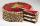Thomas ate 1/3 of cake, Bohouš of the rest of the cake ate 2/5. What fraction of cake left over for others?
• Two divided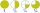Two divided by nine tenths.
• MilimetersHow many millimeters is 1/4 meters?
• Pie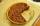Vili ate three pieces of pie . If it piece is 1/8 how much pie did he eat?
• Seeds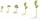Of the 72 wet seeds 7/8 germinated. How many seeds germinate?
• Classroom 4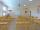In a class of 36 pupils, 2/3 are girls. How much it is in a class girls and boys?
• Women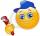In an company of 1050 employees are 2/3 women. How many women work in the company?
• EmployeesOf all 360 employees, there are 11/12 women. How many men work in a company?
• Mixed with percentagesCalculate 33 1/3% of 570.